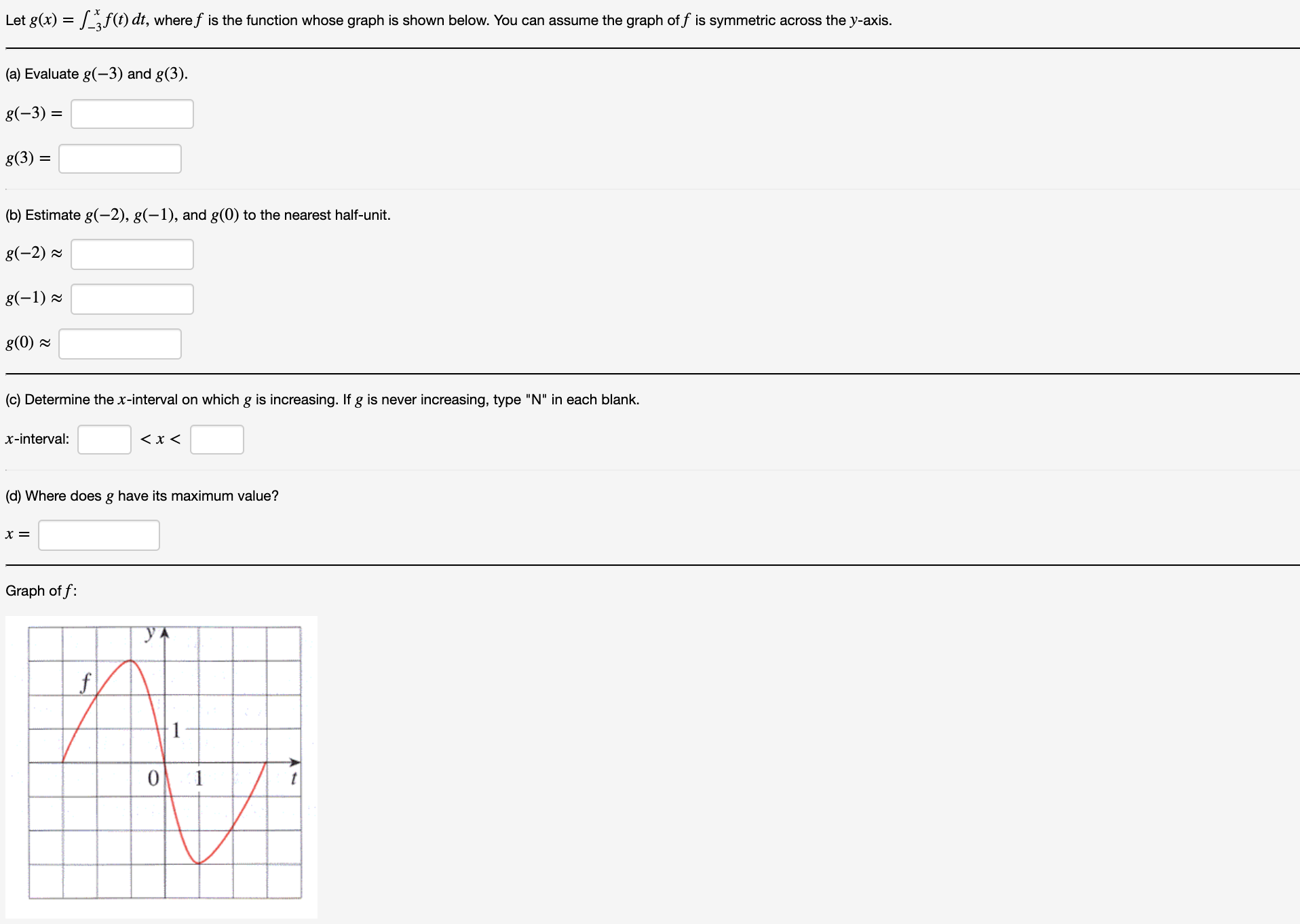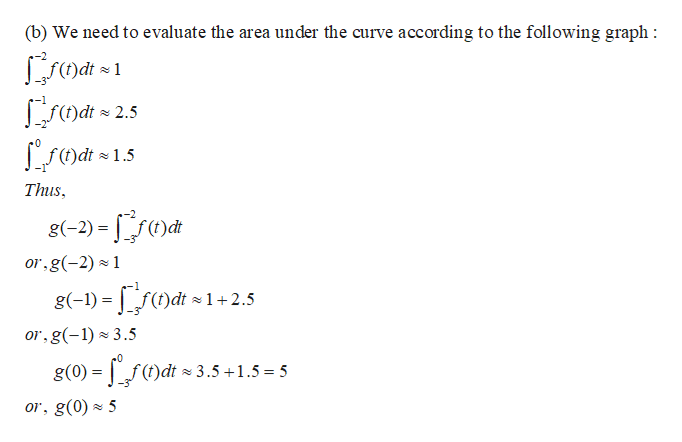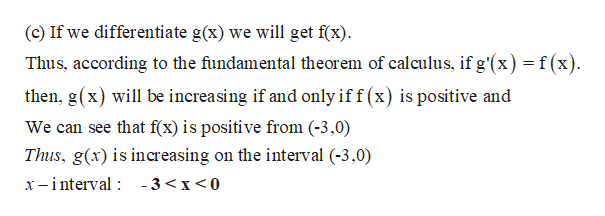# f(t) dt, where f is the function whose graph is shown below. You can assume the graph off is symmetric across the y-axis.Let g(x)(a) Evaluate g(-3) and g(3)g(-3)g(3)(b) Estimate g(-2), g(-1), and g(0) to the nearest half-unit.g(-2)g(-1)g(0)(c) Determine the x-interval on which g is increasing. Ifg is never increasing, type "N" in each blankx-interval< x<(d) Where does g have its maximum value?х%3Graph of f:110

Question
7 viewshelp_outlineImage Transcriptionclosef(t) dt, where f is the function whose graph is shown below. You can assume the graph off is symmetric across the y-axis. Let g(x) (a) Evaluate g(-3) and g(3) g(-3) g(3) (b) Estimate g(-2), g(-1), and g(0) to the nearest half-unit. g(-2) g(-1) g(0) (c) Determine the x-interval on which g is increasing. Ifg is never increasing, type "N" in each blank x-interval < x< (d) Where does g have its maximum value? х%3 Graph of f: 1 1 0 fullscreen
check_circle

Step 1

(a) The figure is shown below:

Step 2

(b)help_outlineImage Transcriptionclose(b) We need to evaluate the area under the curve according to the following graph sdt=2.5 fdt 1.5 Thus g(-2) f or,g(-2) 1 g(-1) f)dt 1+2.5 or,g(-1) 3.5 0 g(0) = f()dt 3.5+1.5= 5 or, g(0) 5 fullscreen
Step 3

(c)

...help_outlineImage Transcriptionclose(c) If we differentiate g(x) we will get f(x) Thus, according to the fundamental theorem of calculus, if g'(x) = f(x) then, g(x) will be increasing if and only if f (x) is positive and We can see that f(x) is positive from (-3,0) Thus, g(x) is increasing on the interval (-3,0) x -interval -3

### Want to see the full answer?

See Solution

#### Want to see this answer and more?

Solutions are written by subject experts who are available 24/7. Questions are typically answered within 1 hour.*

See Solution
*Response times may vary by subject and question.
Tagged in

### Derivative